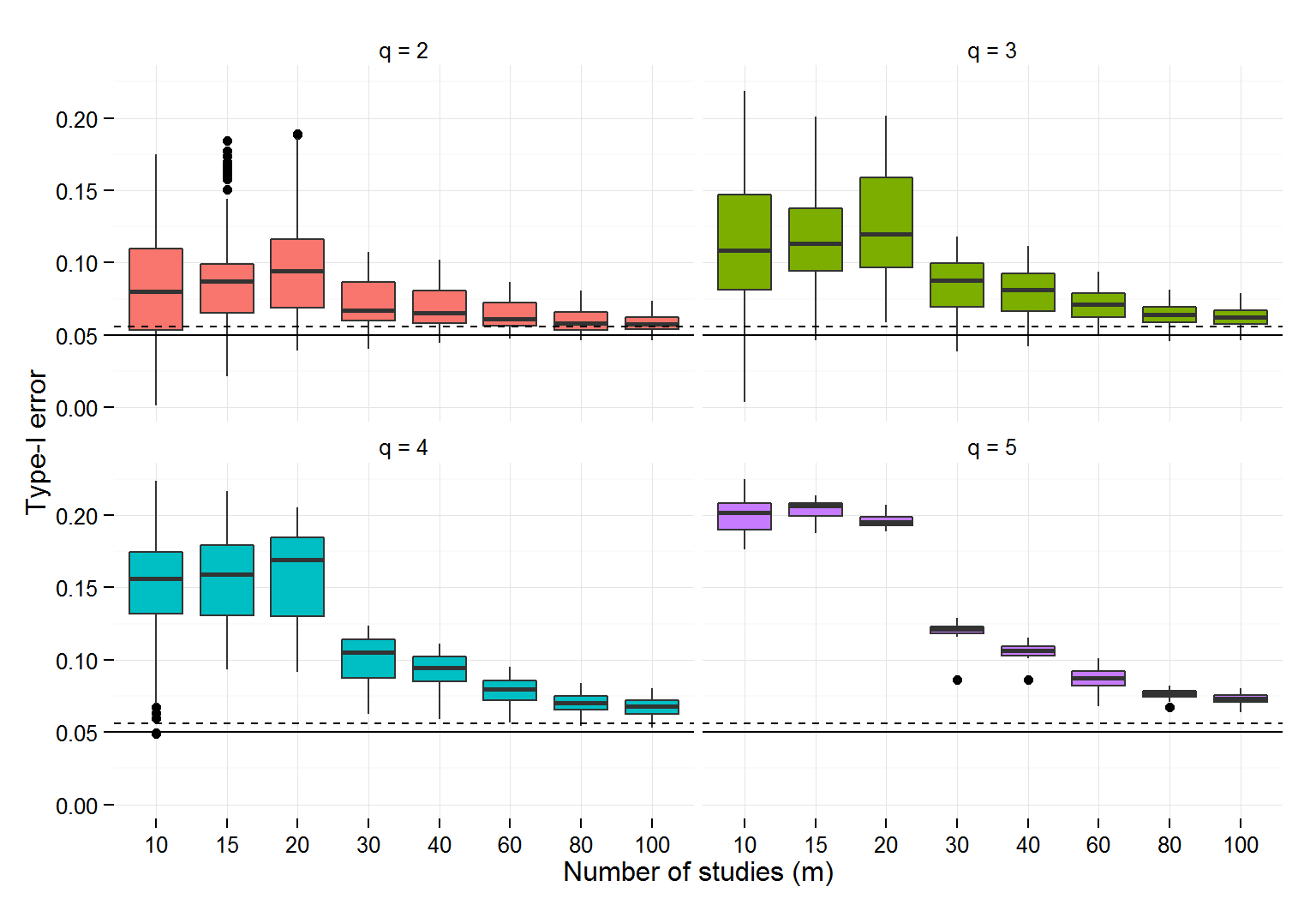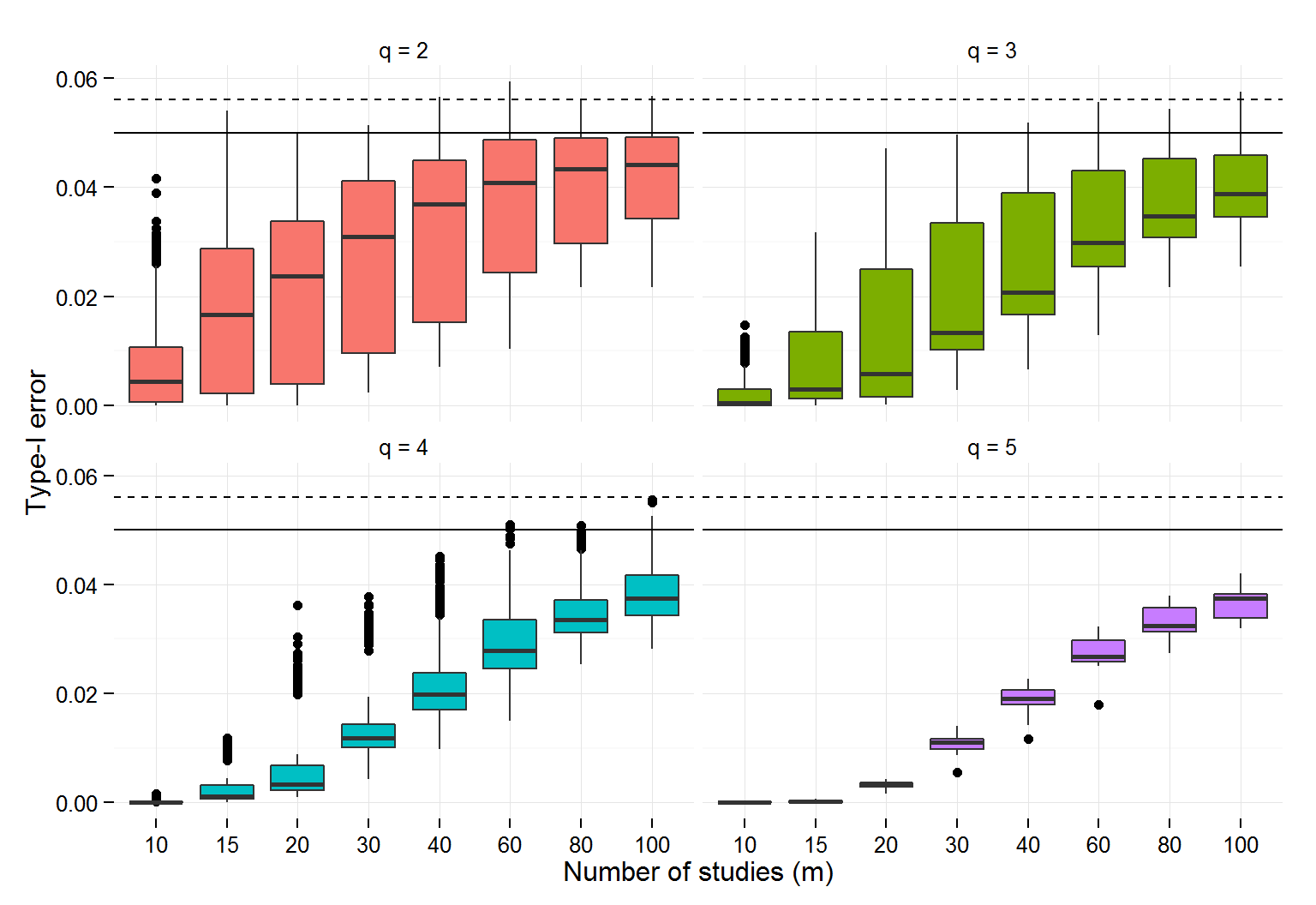March 6, 2015

## Meta-analysis and meta-regression

When one has many intervention studies conducted on a single topic, we may want to pool the results:

• Meta-analysis lets us pools results across studies to obtain estimates of overall efficacy
• For example, "Do the results vary in relation to…"
• Features of the participants in the experiment (e.g., children, teenagers)
• Dosage (e.g., weeks)
• Outcomes measured (e.g., total math, subscale scores, science)
• Study design (e.g., RCT, quasi-experiment)

## Dependent effect sizes

• In meta-analysis, studies often report multiple effect sizes
• Outcomes from multiple tests on the same participants (e.g., math, reading)
• Multiple measures of performance on the same participants (e.g., accuracy, response time)
• Outcomes at multiple time points (e.g., 1-week, 1-month, 1-year)
• Outcomes from multiple experiments (with different participants, but in the same lab)
• Model-based meta-analysis has provided two methods for pooling:
• Univariate meta-analysis, where each study contributes a single effect size, or
• Multivariate meta-analysis, where the covariance structure of the multiple effect sizes is known.
• Neither approach is ideal
• Univariate meta-analysis results in a loss of information
• Multi-variate meta-analysis requires information that is rarely reported in studies.
• In this talk, we will focus on this second approach and its robust alternative.

## Meta-regression model

If each study contributes multiple effect sizes, then the general meta-regression model can be written in vector form: $\mathbf{T}_j = \mathbf{X}_j \beta + \epsilon_j$ for $$j = 1,...,m$$ studies, where

• $$\mathbf{T}_j$$ is a vector of $$n_j$$ effect size estimates from study $$j$$
• $$\mathbf{X}_j$$ is a $$n_j \times p$$ matrix of covariates for study $$j$$
• $$\beta$$ is a vector of $$p$$ meta-regression coefficients
• $$\epsilon_j$$ is a vector of residual errors for study $$j$$ with covariance matrix $$\Sigma_j$$

Given a set of weights, we can estimate $$\beta$$ using weighted least squares: $\mathbf{b} = \mathbf{M} \sum_{j=1}^m \mathbf{X}_j' \mathbf{W}_j \mathbf{T}_j, \qquad \text{where} \qquad \mathbf{M} = \left(\sum_{j=1}^m \mathbf{X}_j' \mathbf{W}_j \mathbf{X}_j \right)^{-1}$

## Model-based meta-regression

Estimating the standard error of $$\mathbf{b}$$ is more difficult.

• If we assume that the weights are inverse variance, with $$\mathbf{W}_j = \Sigma_j^{-1}$$, then $$\text{Var}\left(\mathbf{b}\right) = \mathbf{M}$$.
• This is the multivariate meta-analysis approach, which is "model based."
• It requires correct specification of the covariance matrices $$\Sigma_j$$ and the associated weights $$W_j$$.
• If the true structure of the errors is unknown or mis-specified, then $$\text{Var}\left(\mathbf{b}\right)$$ is wrong.

## Robust variance estimation

Robust variance estimation (RVE; Hedges, Tipton, & Johnson, 2010) produces asymptotically valid estimates of the variance of $$\mathbf{b}$$, even if the error structure is mis-specified.

• RVE uses a "sandwich" estimator: $\mathbf{V}^R = \mathbf{M} \left(\sum_{j=1}^m \mathbf{X}_j' \mathbf{W}_j \mathbf{e}_j \mathbf{e}_j' \mathbf{W}_j \mathbf{X}_j \right) \mathbf{M}$ where $$\mathbf{e}_j = \mathbf{T}_j - \mathbf{X}_j \mathbf{b}$$.

## Hypothesis testing

• In large samples, we can use this variance estimator to construct hypothesis tests. For testing $$\beta_s = 0$$, $z = b_s / \sqrt{V^R_{ss}}$ follows a standard normal distribution if $$m$$ is "big enough."
• In smaller samples, Hedges et al. (2010) suggested that a t-distribution may be more appropriate, with $t = b_s / \sqrt{V^R_{ss}\left(\frac{m}{m-p}\right)}$ compared to a t-distribution with $$m - p$$ degrees of freedom.

## Tests of multiple meta-regression coefficients

• Some hypotheses involve more than one meta-regression coefficient
• Test equality of several levels of a moderator
• Test of overall model fit
• We consider linear hypotheses of the form $\mathbf{C} \beta = \mathbf{c}$ for $$q \times p$$ contrast matrix $$\mathbf{C}$$ and $$q \times 1$$ vector $$\mathbf{c}$$.
• We can construct a Wald test statistic: $Q = \left(\mathbf{C}\mathbf{b} - \mathbf{c}\right)' \left(\mathbf{C} \mathbf{V}^R \mathbf{C}'\right)^{-1} \left(\mathbf{C}\mathbf{b} - \mathbf{c}\right)$
• In large samples, we would expect $$Q$$ to follow a chi-squared distribution with $$q$$ degrees of freedom.
• In smaller samples, an F-test might be better, with
$Q / q \quad \dot{\sim} \quad F(q, m - p)$ But how does this test perform?

## Simulated type-I error rate of F-test## Small-sample corrections

• The originally proposed t-tests have inflated Type-I error with fewer than 40 studies (Hedges et al., 2010; Tipton, 2013, 2014; Williams, 2012).
• Tipton (in press) devised small-sample corrections for t-tests. These corrections involve two parts:
• Adjustments to the variance estimator $$V^R$$
• Estimated degrees of freedom for the t-distribution
• The focus of this paper is on developing similar small-sample methods for F-tests.

## Corrections to the RVE covariance matrix

• Corrections to the RVE estimator based on McCaffrey, Bell, & Botts' (2001) "bias-reduced linearization" approach, using a working model for the error structure: $\mathbf{V}^R = \mathbf{M} \left(\sum_{j=1}^m \mathbf{X}_j' \mathbf{W}_j \mathbf{A}_j \mathbf{e}_j \mathbf{e}_j' \mathbf{A}_j' \mathbf{W}_j \mathbf{X}_j \right) \mathbf{M}$ where the adjustment matrices $$\mathbf{A}_1,...,\mathbf{A}_m$$ are chosen so that $$\text{E}\left(\mathbf{V}^R\right) = \mathbf{M}$$ when the working model is correct.
• Simulation results (for both the t-test and F-test) indicate that the correction helps even if the working model is incorrect.

## Potential corrections for F-tests

• The small-sample t-test developed by Tipton (in press) also adjusted the degrees of freedom.
• These were estimated using a Satterthwaite approximation.
• These degrees of freedom vary in relation to the sample size $$m$$, the number of parameters $$p$$ and features of the covariate.
• By extension, we will look for a degrees-of-freedom correction for F test.
• Drawing on extant literature, we investigated a wide variety of possible corrections.
• Eigenvalue decompositions
• Fai-Cornelius (1996): mixed models
• Cai-Hayes (2008): heteroskedasticity robust standard errors
• Hotellings T-squared approximation
• Zhang (2012, 2013): heteroskedastic ANOVA/MANOVA
• Pan-Wall (2002): generalized estimating equations

## The Winner: $$T^2_Z$$

• The paper provides results for five different corrections. Here, however, we'll focus on only the one that works best.

• The $$T^2_Z$$ approach involves:
• Finding the mean and variance of robust covariance matrix (under a working model)
• Approximating the distribution of robust covariance matrix using a Wishart distribution
• Matching the mean and total variance of the robust covariance matrix to estimate the Wishart degrees of freedom
• $$Q/q$$ tested against Hotelling's $$T^2$$ distribution
• The $$T^2_Z$$ is best in two regards
• It is (almost) always level-alpha
• It is more powerful than any of the other level-alpha estimators (i.e., always has error rates closer to nominal)

## Simulation results: $$T^2_Z$$## Example: Wilson et al. (2011)

• Wilson, Lipsey, Tanner-Smith, Huang, & Steinka-Fry (2011) synthesis of effects of dropout prevention/intervention programs.
• Primary outcomes: school completion, school dropout
• $$m = 152$$ studies, containing 385 effect size estimates
• Some studies included effect sizes for multiple outcomes, measured on the same sample
• Some studies include effect sizes from multiple samples
• Meta-regression model including several categorical moderators
• Study design: 3 levels (non-experimental, matched groups, randomized experiment)
• Outcome measure: 4 levels (school enrollment, dropout, graduation, graduation or GED)
• Evaluator independence: 4 levels (involved in delivery, involved in planning, indirect involvment, independent)
• Implementation quality: 3 levels (clear problems, possible problems, no apparent problems)
• Program format: 4 levels (community-based, classroom-based, school-based, multiple formats)

## Wilson et al. (2011) test results

Moderator q Naive F p-value T-squared Z d.f. p-value
Study design 2 0.23 0.796 0.22 43 0.800
Outcome measure 3 0.91 0.436 0.84 22 0.488
Evaluator independence 3 3.11 0.029 2.78 17 0.073
Implementation quality 2 14.15 <0.001 13.78 37 <0.001
Program format 3 3.85 0.011 3.65 38 0.021

Naive F test uses $$m - p = 130$$ degrees of freedom.

## Conclusions and future work

• Like Tipton (in press) found with the small-sample t-test…
• The performance of the large-sample test depends on features of the underlying covariate properties.
• Consequently, it is hard to know a priori what constitutes a "big enough" sample.
• We therefore recommend that small-sample corrections should always be used in practice.
• We provide prototype software in R (upon request), and are working on implementing it fully into the robumeta R package and Stata macro and the metafor R package (Viechtbauer, 2010).
• Future work
• Investigate power of tests based on RVE versus model-based methods.
• Investigate other areas of application beyond meta-analysis, including
• Hierarchical linear models
• Econometric panel data models